cum
top of page
Search

# CAPE Unit 1 Physics: Motion Part 1 (Linear and Projectile)

This post will cover Unit 1, Module 1, Section 3, Parts 1-7.

First, let's look at a couple terms necessary to know before we tackle motion.

## Key Definitions

Displacement- Displacement is a vector quantity representing the magnitude (size) and direction of the shortest path between two points.

Remember: Vector quantities have both magnitude and direction, while scalar quantities only have magnitudes.As you can see, displacement is simply the straight line directional space between A and B, while distance, a scalar quantity, encompasses all of the contradictory turns taken between the two points, regardless of whether the net movement is the same or the direction of the movement.

Speed- Speed is a scalar quantity representing the distance travelled per unit time (SI Unit m/s).

Instantaneous vs Average Speed

If you check the odometer of a vehicle in motion, you will se the instantaneous speed, or the value of speed at that exact point in time (this can be determined as the gradient of a distance-time graph at a specific point). However, if you were to measure the total distance travelled by a vehicle over a journey and then divide by the total time taken to complete the journey, the result would be the average speed of the vehicle throughout the journey.

Velocity- Velocity is a vector quantity defined as the displacement per unit time (SI Unit also m/s, but as a vector quantity, it has a direction).

Acceleration- Acceleration is the change in velocity per unit time (SI Unit ms^-2). Note that it is a vector quantity, but we are usually only concerned with the magnitude of acceleration.

Acceleration can be determined as:Where v is final velocity, u is initial velocity and t is the time throughout which the acceleration (or deceleration occurs).

## Graphing Displacement, Speed, Velocity, and Acceleration

Most frequently when it comes to motion, you'll see graphs of one of the above quantities versus time. These graphs are useful for determining the values of the other quantities.

Note: These graphs will only represent motion in a single dimension (i.e. one dimensional motion).

Displacement-Time Graphs

The velocity can be determined as the gradient of a displacement-time graph.

The total displacement will just be the final value of displacement on the graph (y-value of the last point on the graph).The graph above shows a body travelling at a constant velocity (the quantity is velocity since the graph is displacement versus time rather than distance versus time).The graph above shows a body at rest (velocity of zero). Consequently, the gradient of the graph is also zero.This graph shows a body accelerating uniformly. The gradient at a certain point of the graph will give the velocity at the specific time.

Velocity-Time Graphs

The acceleration of an object can be determined as the gradient of a velocity-time graph.

The total displacement can be calculated as the area under a velocity-time graph. For example, the graph below forms a triangle with the x-axis, meaning that the area beneath is could be calculated as bh/2 (b-base, h-height). The base would be the total time while height would be final velocity. Other graphs may form trapezoids or more complex shapes, requiring other formulae as needed.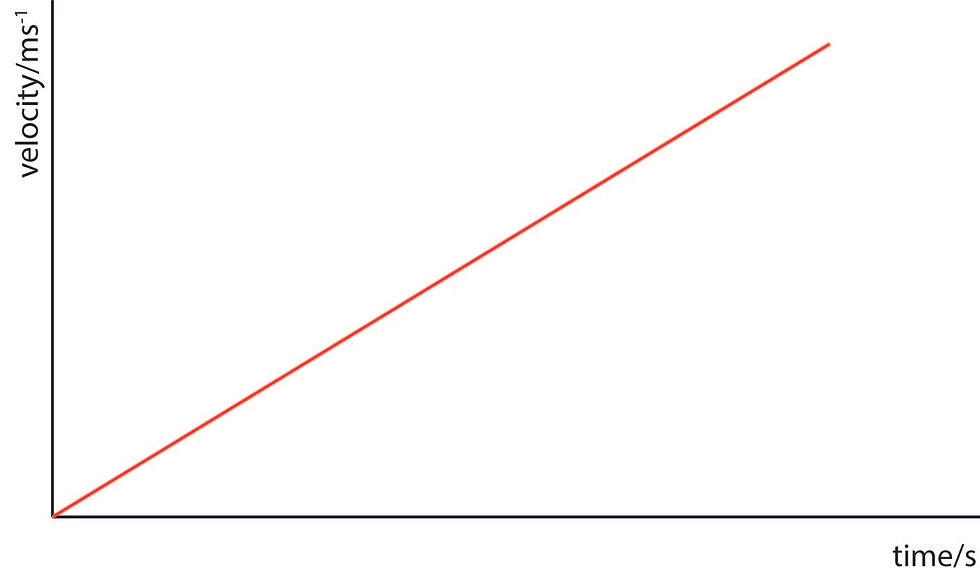The graph above shows an object accelerating uniformly from rest.This graph is of an object decelerating uniformly.This graph is of an object moving at uniform velocity.This graph is of an object whose acceleration is increasing from rest.

Acceleration-Time Graph

The velocity of an object will be the area under an acceleration time graph. It's unlikely that you'll encounter acceleration time graphs of gradients that aren't zero.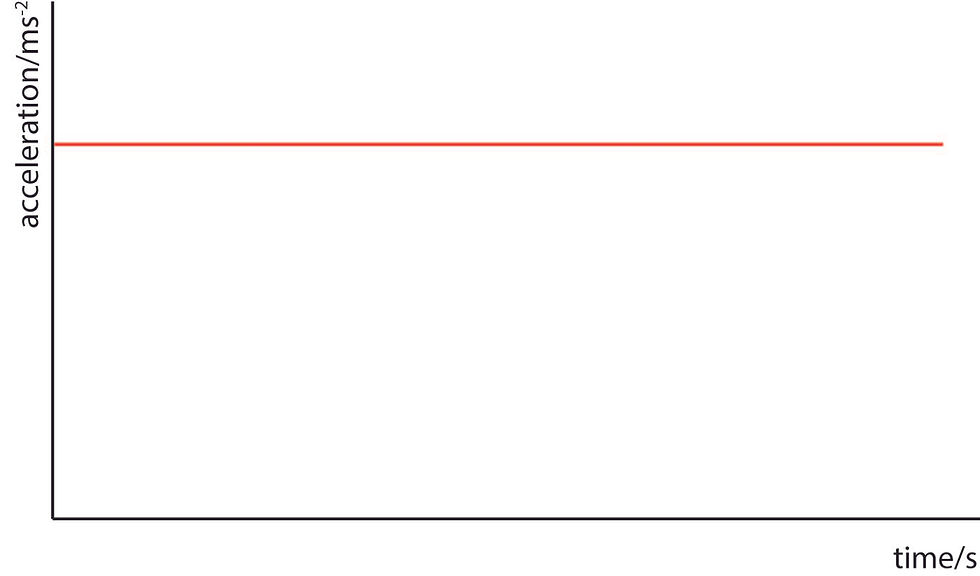The graph above shows an object accelerating uniformly.

## The Kinematic Equations (and their Derivations)Soon, we'll meet 5 of your best friends for the entire topic of motion- the kinematic equations! (You now have permission to celebrate). First, let's think about an arbitrary velocity time graph, showing an object which accelerates from an initial velocity (known as u) to a final velocity (known as v) over a time (t):The graph takes the form of a trapezium. To determine the displacement of the object, we simply find the area under the graph. Since the area of a trapezium is found as:And the Area (A) is the displacement (s), a can be said to be u, b can be v, and h can be t:And there's your first kinematic equation! We'll use this to derive all the others, along with the equation for acceleration, which, if you remember, is:If we transpose the equation for v (i.e. make v the subject by performing operations), we'll see another kinematic equation:By substituting the second equation into the first, we can determine our next kinematic equation:The final kinematic equation can be derived by rearranging a previous equation for the value of t, the substituting this value of t into the equation above:Then, multiplying through by a:Dividing by a half (multiplying by two) allows us to get rid of the final fraction:And there is the final horseman of the kinematic apocalypse.

(Note: These equations can only be used to solve problems in which acceleration is uniform or constant)

## Linear Motion

In the forms given above, these equations are used in the solution of linear motion problems (movement of objects in a straight line), for example, a ball falling in a straight line, or any vehicle travelling in a straight path.

Note: the value of acceleration in your linear motion calculations will be reliant on whether the motion is vertical (affected by acceleration to gravity, 9.81 m/s^2) or horizontal (not affected by gravity).

Let's look at the example of a bouncing ball.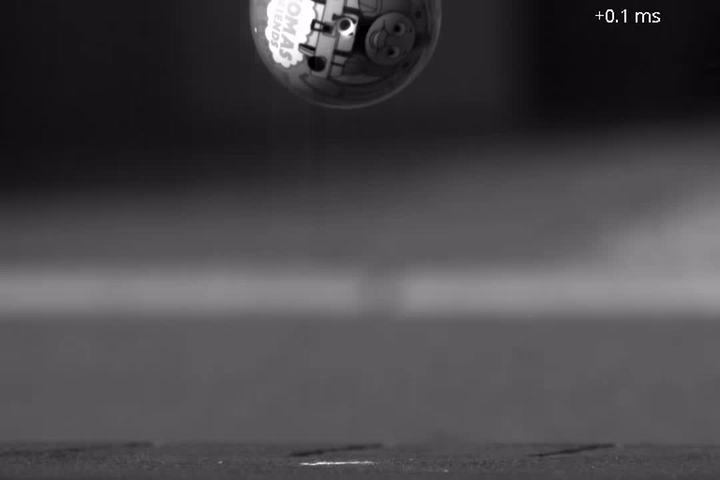In a perfect system, a ball follows a straight line path from where it's dropped to the ground, influenced only by the acceleration due to gravity. When it makes contact with the ground, it quickly slows to a halt, before rebounding to a lower height than that it was dropped from. We can draw a velocity-time graph to illustrate this motion:If we take movement downwards towards the ground as positive displacement and movement upwards as negative displacement, the resulting velocities will be positive and negative respectively.

At each bounce, the direction of movement switches, which is why those straight lines are shown. These lines don't necessarily have to be straight; since the change in direction is not instantaneous, they may have a gradient showing the deceleration as the ball changes direction.The acceleration-time graph would appear as follows:Note that the acceleration becomes negative after the bounce, since gravity continues to act downwards, in an opposite direction to the movement of the ball.

## Projectile MotionQuite obviously, objects don't always just move in straight lines. What about the path travelled by a ball when thrown?A ball dropped will follow a linear path and therefore its motion can be described with our linear motion equations. However, a ball thrown sideways will require our fancy projectile motion equations.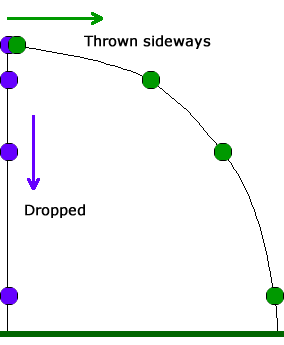Let's say, for example, that there is a ball being thrown:At each point along its path, the ball has a velocity acting at an angle to the horizon which is related to the initial velocity with which it was thrown and acceleration due to gravity (considerations of air resistance and the like are not included).

Whenever we have forces/vectors acting in several directions like this, we pretty much always resolve these forces into horizontal (x) and vertical (y) components.Given the value of the resultant velocity, we can find the horizontal and vertical components like this:As long as the vectors occupy this form, the formulae will be the same.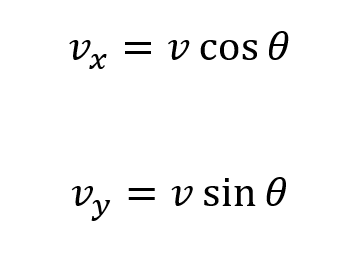Now, given that we must now resolve the velocity vector into horizontal and vertical components, we must also adjust our kinematic equations for dealing with horizontal and vertical components separately.

### Horizontal Components in Projectile Motion

We'll look at the horizontal components first (because they're easier). Let's take a look at our first kinematic equation (although the order really doesn't matter):v, our final velocity, will instead be the horizontal component of v, and u (initial velocity) will be the horizontal component of u. a will have a value of zero since gravity only acts vertically, and we won't be considering forces like air resistance. Simplifying:Since no acceleration is considered to affect the system, the horizontal initial velocity will not change throughout the motion of the object. Since we know this, the only other equation we can use is:Substituting and simplifying:This value of s is the horizontal displacement of the object in motion. This can be represented as range, R.### Vertical Components in Projectile Motion

As acceleration acts vertically, a will be taken as acceleration due to gravity (represented as g). Since gravity acts downwards, i.e. in a direction opposite to the vertical component of velocity, it will be taken as negative. Let's start by substituting the vertical components into the kinematic equations:### Useful Equations in Projectile Motion

It's common for questions to ask about the maximum height reached by an object. At the maximum height, or apex point, you'll notice that the resultant velocity is only horizontal, or parallel to the horizon:This means that at the maximum height, the velocity is zero. Thus, if we use one of the kinematic equations, we can transpose to find s, or the vertical displacement (height) when vertical velocity is zero:Maximum height is represented as hm here.

Note: This formula will give the maximum height above the point it was thrown from.

The time taken to react maximum height can also be calculated:The time taken to reach maximum height is represented as t↑.

The total time of flight, however depends on the way in which the projectile motion occurred.

## Cases of Projectile Motion

Projectile motion can occur in several ways, each of which you'll be able to discern based on how the question is posed.

First CaseThe object in motion moves from the ground and returns to ground level.The maximum height is the same as the equation given earlier, and the total time of flight is two times the time taken to reach max height: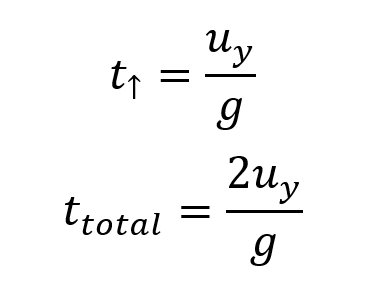Second CaseThe object is thrown up and off of an elevated platform. The maximum height will be the previous equation plus the initial height of the object, or the height of the platform:Thus, the time taken to fall must also include the additional height. It will therefore be the time taken to reach the maximum height added to the time taken to reach the ground from the maximum height:But, since we are taking the initial velocity as the vertical velocity at max height, initial velocity is zero: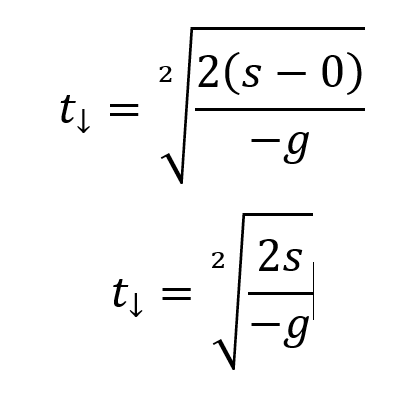Note that the value of g is shown as negative here. This is because the height is seen as 'negative' now that our initial velocity is at the max height and has a value of zero. Therefore, the equation can be written more simply:Total time of flight is therefore:Third CaseThe object is thrown sideways off of an elevated platform such that the initial velocity is parallel to the horizontal. Thus the max height is the same as the initial height. The total time of flight is only the time taken to descend from the max height, which we saw previously:## Projectile Motion... Is Parabolic

If you're familiar with quadratic equations, you'll know that they take the form:Where a, b and c are constants. The graphs of quadratics are parabolas:The maximum parabola above looks a lot like the paths of motion we have been illustrating with the previous diagrams. Now we'll look at proving mathematically that they are one in the same.

Let's propose that s (displacement) represent position on the x and y axes:

x-axis:On the y-axis:We can transpose the equation of x-position for t:Substituting into the equation for y-position:You can see that the result is a quadratic equation! If we want to get really fancy, we can substitute in the values of ux and uy:Thanks for reading! Be sure to send any questions you have!

1,181 views1 comment

## Recent Posts

See All

Hey Chemistry warriors! Here's a quick support paper for those of you doing Unit 1 this year. Lots of useful questions covering the...

bottom of page